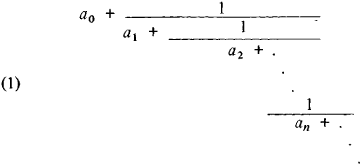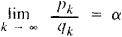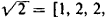# Continued Fraction

(redirected from Continued fractions)
Also found in: Dictionary, Thesaurus, Acronyms.

## continued fraction

[kən′tin·yüd ′frak·shən]
(mathematics)
The sum of a number and a fraction whose denominator is the sum of a number and a fraction, and so forth; it may have either a finite or an infinite number of terms.

## Continued Fraction

one of the most important ways of representing numbers and functions. A continued fraction is an expression of the formwhere a0 is any integer and a1, a2, . . . , an, . . . are natural numbers, called the partial quotients of the given continued fraction.

We can arrive at the continued fraction representing some number α by writing this number in the form α = a0 + (l/α1), where a o is an integer and 0 < 1/α1 < 1; we then write a1 in this form, and we continue in this manner. The number of quotients of a continued fraction may be finite or infinite, and the continued fraction is correspondingly called finite or infinite. The continued fraction (1) is often denoted symbolically as

(2) [a0;a1,a2, ... , an, ... ] infinite continued fraction or

(3) [a0;a1, a2, ... , an] finite continued fraction

A finite continued fraction is always a rational number; conversely, every rational number can be represented in the form of a finite continued fraction (3). Such a representation is unique if we require that an ≠ 1. The continued fraction [a0; a1, a2, . . . , ak (k ≤ n) written in the form of an irreducible fraction Pk/qk is called the kth convergent of the continued fraction (2). The numerators and denominators of convergents of continued fractions are related by the recursion formulas

Pk + 1 = ak + 1Pk + Pk - 1 and qk - 1 = ak + qk + qk - 1

which serve as the foundation for the entire theory of continued fractions. These formulas directly imply the important equation

pkqk + 1 − qkpk1 = ± 1

For every infinite continued fraction, there exists a limitcalled the value of the given continued fraction. Every irrational number is the value of a unique infinite continued fraction that can be obtained by expanding α in the above manner; for example, (e - l)/2 = [0, 1, 6, 10, 14, 18, ... ] and= [1, 2, 2, . . . ]. Quadratic irrationalities yield periodic continued fractions.

The chief value of continued fractions for applications is that the convergents of a continued fraction are the best approximations of a number α in the sense that for any other fraction m/n whose denominator is at most qk we have the inequality |na —m\ qkα − pk |. Here,|qk a —pk < 1/qk + 1- The odd convergents of a continued fraction are greater than α, and the even convergents are less than α. With increasing k, the odd convergents of a continued fraction decrease and the even convergents increase.

Continued fractions are used to approximate irrational numbers by rational numbers. For example, the well-known approximations 22/7 and 355/113 for the number π (ratio of the circumference of a circle to the diameter) are convergents of the continued fraction expansion of π. It should be noted that the first proof that the numbers e and Π are irrational was given in 1766 by the German mathematician J. Lambert using continued fractions. The French mathematician J. Liouville proved that for any algebraic number α of degree n it is possible to find a constant λ such that |a —x/y | > λ/yn for any fraction x/y. By means of continued fractions, it is possible to construct numbers a such that the difference |α —pk/qk| can be made less than λ/qk for an arbitrary choice of the constant λ. It is thus possible to construct transcendental numbers by using continued fractions. A major drawback of continued fractions lies in the extraordinary difficulty (in practice, the impossiblity) of performing arithmetic operations on them. For example, given the elements of two continued fractions, we have no simple way of obtaining the elements of their sum or product.

Continued fractions had already been encountered in the 16th century in the work of R. Bombelli. In the 17th century, continued fractions were studied by J. Wallis, and a number of important properties of continued fractions were discovered by C. Huygens, who investigated them in connection with the theory of gears. In the 18th century, L. Euler made many contributions to the theory of continued fractions.

In the 19th century, many researchers, including P. L. Chebyshev and A. A. Markov, applied continued fractions whose elements are polynomials to the study of orthogonal polynomials.

### REFERENCES

Chebyshev, P. L. Polnoe sobranie sochinenii, 2nd ed., vol. 1. Moscow-Leningrad, 1946.
kinchín. A. Ia. Tsepnye drobi, 2nd ed. Moscow-Leningrad, 1949.
Euler, L. Vvedenie v analiz beskonechno malykh, vol. 1. Moscow-Leningrad, 1936. (Translated from Latin.)
Stieltjes, T. J. Issledovaniia o nepreryvnykh drobiakh. Kharkov-Kiev, 1936. (Translated from French.)
Perron, O. Die Lehre von den Kettenbrüchen, 2nd ed. Leipzig-Berlin, 1929.
Wall, H. S. Analytic Theory of Continued Fractions. Toronto-New York-London, 1948.
References in periodicals archive ?
We give 2-, 4-, 8- and 16-dissections of a continued fraction of order sixteen.
Caption: Figure 5: Physical implementation of dynamical characteristics in the form of a continued fraction sum.
As in the classical context of real numbers, we have a continued fraction algorithm in K(([X.
In a previous paper , for any positive integer we introduced the notion of real quadratic fields with period l of minimal type (see Definition below) by using the simple continued Fraction expansions with period l of certain quadratic irrationals, and proved that there exist exactly 51 real quadratic fields of class number 1 that are not of minimal type, with one more possible exception ([3, Proposition 4.
A continued fraction can be obtained by iterating such transformations.
It is my hope that Philippe (as i am allowed to call him) will like my own research on continued fractions as well.
1975), "Properties of continued fractions with applications in Markov processes", J.
Since the continued fraction will give the value of a ratio [f.
For a = 2, 3,4 and [p - 1]/2, from the above Theorem and the definition of continued fractions we may immediately obtain the following four Corollaries.
Attempts to transform the asymptotic series to a continued fraction by the qd algorithm do not work, which is another common problem for asymptotic series with coefficients of one sign.
All quadratic surds have continued fractions in which the terms repeat after a certain point, i.

Site: Follow: Share:
Open / Close